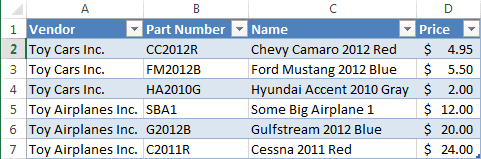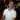# How to use Index with Match to lookup values

August 28, 2012

You don’t really want to use Vlookup, do you? Why? It’s so limited compared to using Index with Match. Index with Match is so much more flexible than using Vlookup that I actually NEVER use Vlookup after I learned Index with Match in Excel.

Let’s look at the following:What would you say if I were to ask you “Please give me the part number for ‘Chevy Camaro 2012 Red’.“?

You may try to use the Vlookup function like this:

``=VLOOKUP("Chevy Camaro 2012 Red",\$B\$1:\$C\$7,1,FALSE)``

However, this formula results in `#NA!` which we don’t want.

This is one of the problems with Vlookup: you have to match your table layout to what the function expects. One possible solution to this problem could be to switch columns C and B.  But really, who wants to change their table just because a function wants it that way?

Another solution is to use Index with Match. Let’s take an in-depth look at how to use the Index with Match functions.

## How Index with Match Works

To start, here’s how you would get the Part Number from the table based on the Name of the toy:

``=INDEX(\$B\$1:\$B\$7,MATCH("Chevy Camaro 2012 Red",\$C\$1:\$C\$7,0),1)``

This returns `CC2012R`, which we want. Let’s dig into the formula to figure out how this works.

As I said before about Excel Formulas, they work from the inner-most item that can be evaluated and then works its way outwards. Since we have the `MATCH()` function inside `INDEX()` function like `=INDEX(range,MATCH(text,range,1),1)`, it makes sense to start with the Match function and how that works, then we’ll look at the Index function, and then how it all ties together.## Match Function Basics

The Match function looks at a range of contiguous cells for a certain value and then returns the relative row/column number of its position within the range. It has the form of:

``=MATCH(lookup_value, lookup_array, [match_type])``

Here is a simple explanation of each parameter:

Parameter Notes
`lookup_value` This is the number or text that you’re looking for. This can be a straight value or a cell reference.
`lookup_array` cell reference of the table range. This must be either 1 column wide with many rows or 1 row high with many columns.
`[match_type]` This parameter is optional with a default value of 1. It basically tells the Match function how you want to find the data (I’ll get more detailed about this in a minute).

The `[match_type]` parameter can be 1 of 3 choices:

Type Notes
1 Less Than This option tells the function to find a match from the lookup_value that is as less than or equal to the value being searched. For example, if you’re looking for a number like 100 and you have numbers 1-99 and 101 (but not 100), it will return 99 since it is the closest, yet less than, 100. For text, it searches for a value that is alphabetically less than or equal to what you’re searching for. NOTE: the values in the lookup_array must be sorted from least to greatest for this option to work correctly.
0 [Exact Match] This option tells the function to find an exact match in the lookup_array for the lookup_value.
-1 [Greater Than] This option is the polar opposite of the [Less Than] option. It looks for the smallest match that is greater than or equal to the lookup_value passed. So in our previous example where you are searching for 100 but you have only numbers 1-99 and 101 (but not 100), then it will return 101. NOTE: the values in the lookup_array must be sorted from greatest to least in order to work correctly.

OK, now that we have a basic understanding of how this function works, let’s take a look at how we applied it to our question:

``=MATCH("Chevy Camaro 2012 Red",\$C\$1:\$C\$7,0)``

And here is our table again for easy reference:Based on the formula’s parameters, we are looking for an exact match against the range `\$C\$1:\$C\$7` where is has the text “Chevy Camaro 2012 Red”. The result comes back with `2`, which is the relative row number in our range. This row number will be used later as we’ll see in the Index function.

As a side note, you can use the Match function to also determine the column number of a range. For example, if you want to find what column “Part Number” is in, you would use this formula:

``=MATCH("Part Number",\$A\$1:\$D\$1,0)``

This would return `2`.

## Index Function Basics

The Index function takes a range of cells and then hones in on one specific cell within the range. The formula is defined as:

``=Index(array, row_num, column_num)``

Here is a simple explanation of each parameter:

Parameter Notes
`array` This is the cell reference of the table range that you want to get your return value from. This can be as small or big as you want with as many columns/rows as you need.
`row_num` This is the row number you want to reference from the array passed.
`column_num` This is the column number you want to reference from the array passed.

If we knew which cell we wanted to select from the range passed, an easy Index formula would look like:

``=INDEX(\$B\$1:\$B\$7,2,1)``

So from the range of Part Numbers, I want to return the cell in the second row and the first column. This would return `CC2012R`. Easy, right? In this case, yes, it is. However, we would hardly ever know which row/column we want, which is why we need the help of the Match function.

## How Index and Match Work Together

By now, it should start making sense how these two functions work together. The Match function is used to determine the relative row number or column number (or both). From there, we take those values and target a specific cell in the range that was passed to the Index function. For our formula, Match is returning the row number that matches the “Name” column. And in the end, we get this:

``=INDEX(\$B\$1:\$B\$7,MATCH("Chevy Camaro 2012 Red",\$C\$1:\$C\$7,0),1)``

### Wait, wait…why are you not passing in the whole table for the range?

Great question! You could pass the entire table’s range, but it’s not necessary. It’s based on relative referencing. That’s the beauty of using Index with Match. We need to return a value from column B based on a match in column C which is why all Index needs to care about is column B and Match only needs to care about column C.

There is one caveat, though, which is that the amount of rows and columns for the Index array should match with what the Match functions have used inside the `row_num` and `column_num` parameters, respectively. So if I excluded the headers from the Index array, but not the Match function, we’ll get an error:``=INDEX(\$B\$2:\$B\$7,MATCH("Chevy Camaro 2012 Red",\$C\$1:\$C\$7,0),1)``

This returns `FM2012B`, which is incorrect. This is because the Index array starts at `\$B\$2` and the Match function starts at `\$C\$1`. So when Match comes back with `2`, `2` for Index is something else (in this case, `FM2012B`). Just remember to make sure the relative ranges will coincide with each other and you’ll be fine.

I hope you enjoyed this post and if you did, please subscribe! You will get notified of newer posts the moment they are published. Also, if you have any questions or comments, or if you want to see me post about something, please leave a comment below. It really helps me to make this blog better and I would love to hear from you.

Wow, you read the whole article! You know, people who make it this far are true learners. And clearly, you value learning. Would you like to learn more about Excel? Please consider supporting me by buying me a coffee (it takes a lot of coffee to write these articles!).Written by Joseph who loves teaching about Excel.